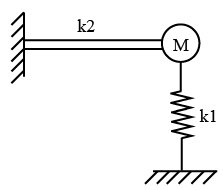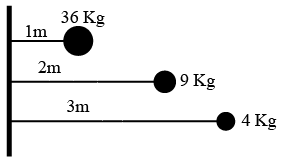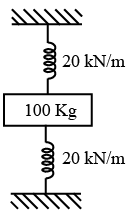# ISRO Scientist or Engineer Mechanical 2006

Instructions

For the following questions answer them individually

Question 11

# A mass m attached to a light spring oscillates with a period of 2 sec. If the mass is increased by 2 kg, the period increases by 1 sec. The value of m isQuestion 12

# A short column of external diameter D and internal diameter d carries an external load W. The greatest eccentricity which the load can have without producing tension on the cross section of the column isQuestion 13

# If the radius of wire stretched by a load doubled, then its Young’s modulus willQuestion 14

# Longitudinal stress in a thin cylinder subjected to internal pressure isQuestion 15

# Maximum deflection in cantilever due to pure bending moment M at its end isQuestion 16

# If Poisson’s ratio for a material is 0.5, then the elastic modulus for the material isQuestion 17

# A cantilever beam of negligible weight is carrying a mass at its free end, and is also resting on an elastic support of stiffness $$k_1$$ as shown in the figure below.If $$k_2$$ represents the bending stiffness of the beam, the natural frequency (rad/s) of the system isQuestion 18

# The figure shows 3 small spheres that rotate about a vertical axis. The perpendicular distance between the axis and the center of each sphere is given. Mass of highest rotational inertia about that axis, isQuestion 19

# Which of the following relationships between the force F on a particle and the particlees position x implies simple harmonic oscillationQuestion 20

# A mass of 100 kg is held between two springs as shown in figure. The natural frequency of vibration of the system in cycles/second isOR